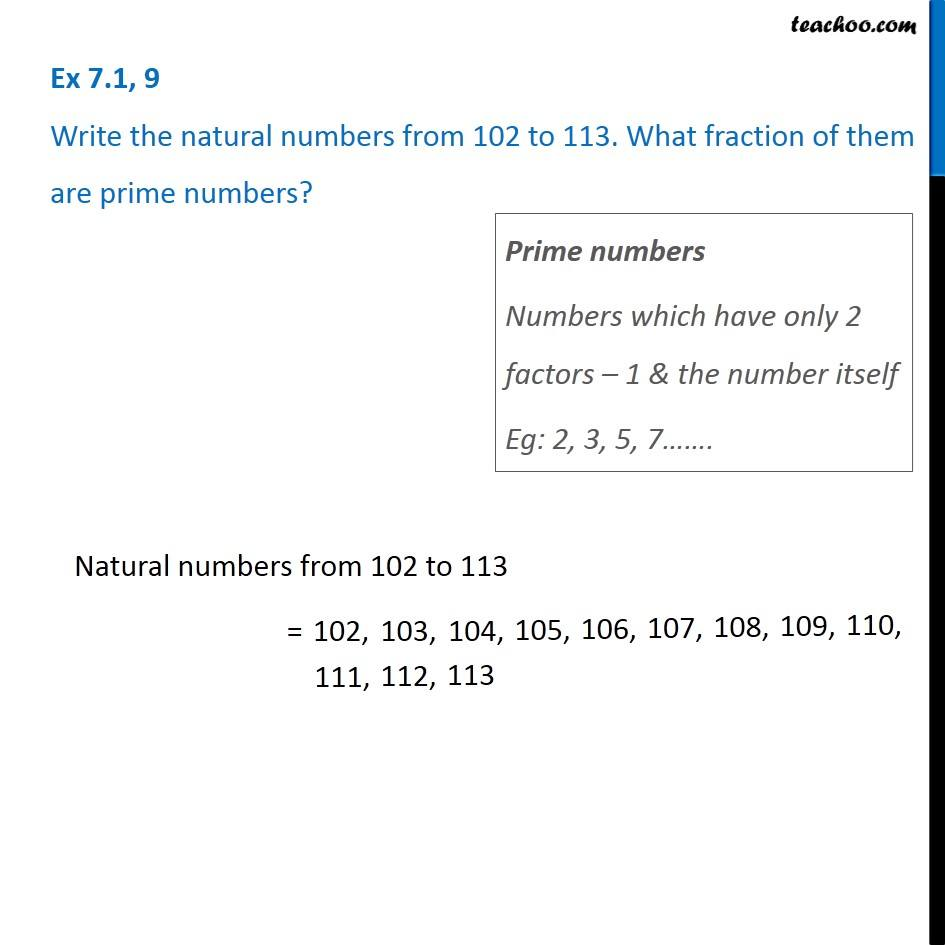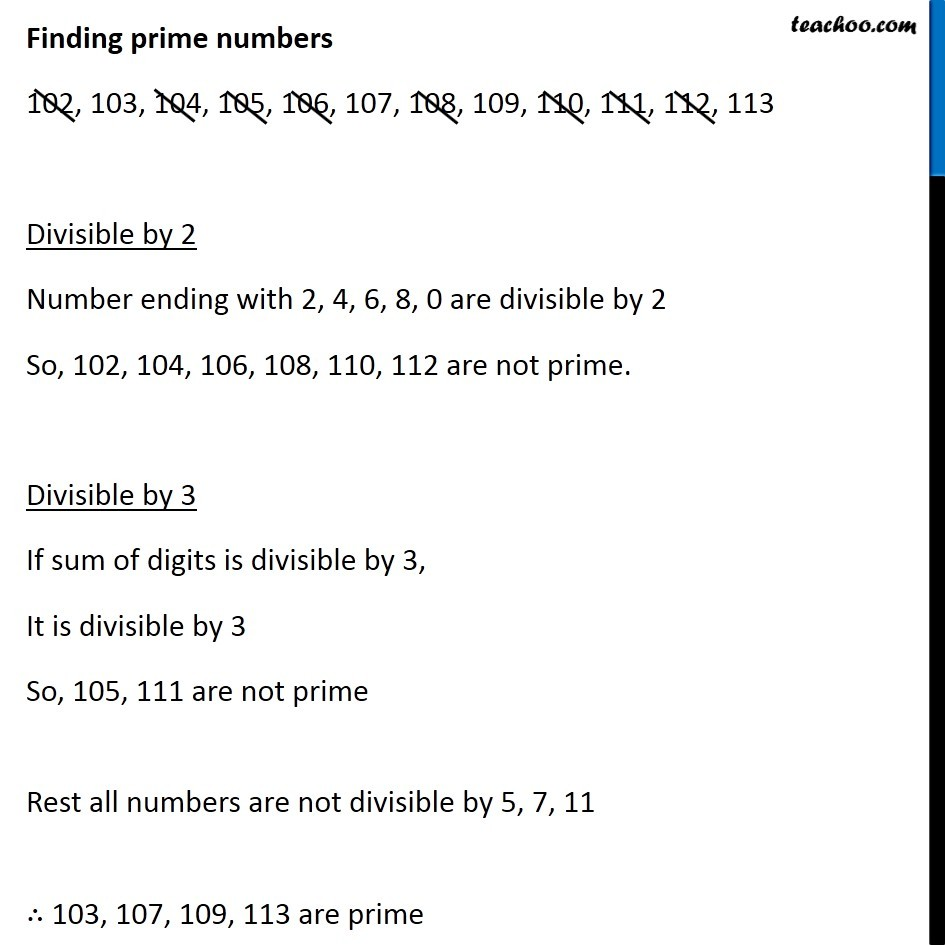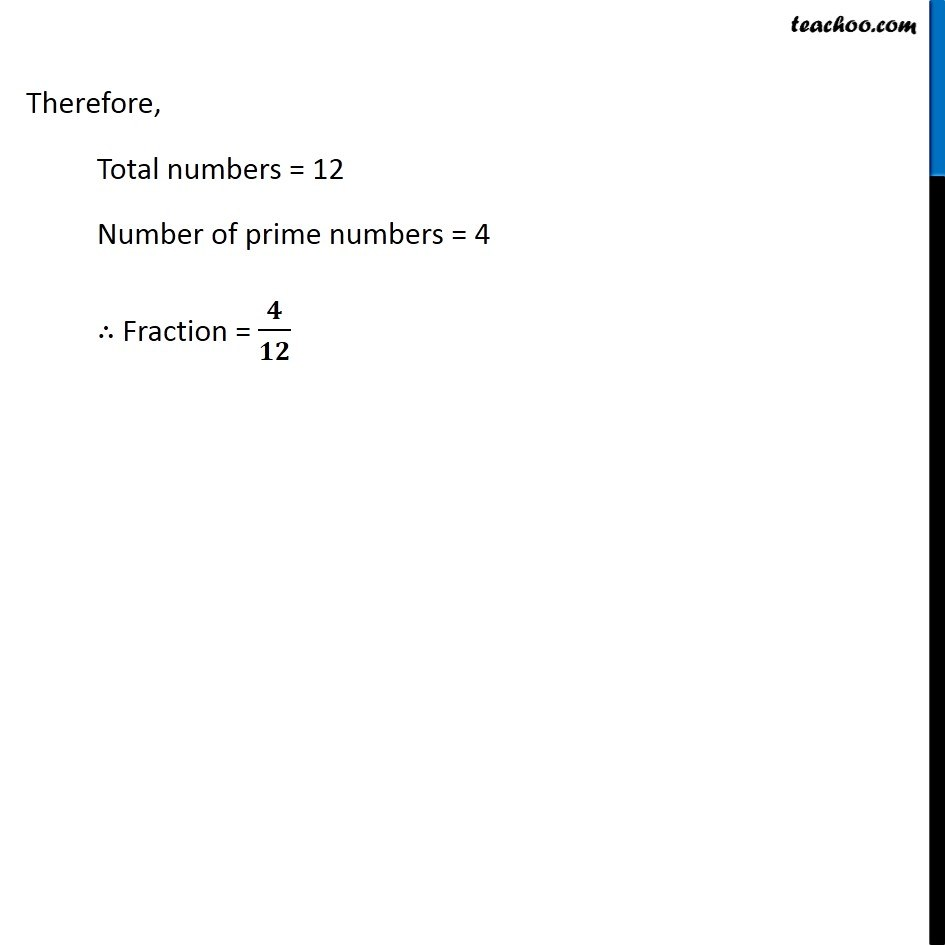Ex 7.1

Chapter 7 Class 6 Fractions
Serial order wiseLearn in your speed, with individual attention - Teachoo Maths 1-on-1 Class

### Transcript

Ex 7.1, 9 Write the natural numbers from 102 to 113. What fraction of them are prime numbers? Prime numbers Numbers which have only 2 factors – 1 & the number itself Eg: 2, 3, 5, 7……. Natural numbers from 102 to 113 = Finding prime numbers 102, 103, 104, 105, 106, 107, 108, 109, 110, 111, 112, 113 Divisible by 2 Number ending with 2, 4, 6, 8, 0 are divisible by 2 So, 102, 104, 106, 108, 110, 112 are not prime. Divisible by 3 If sum of digits is divisible by 3, It is divisible by 3 So, 105, 111 are not prime Rest all numbers are not divisible by 5, 7, 11 ∴ 103, 107, 109, 113 are prime Therefore, Total numbers = 12 Number of prime numbers = 4 ∴ Fraction = 𝟒/𝟏𝟐